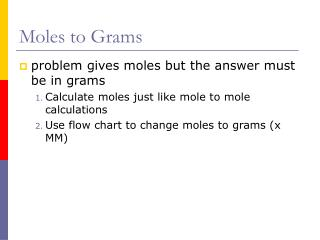DownloadDownload PresentationMoles to Grams

# Moles to Grams

Télécharger la présentation## Moles to Grams

- - - - - - - - - - - - - - - - - - - - - - - - - - - E N D - - - - - - - - - - - - - - - - - - - - - - - - - - -
##### Presentation Transcript

1. Moles to Grams • problem gives moles but the answer must be in grams • Calculate moles just like mole to mole calculations • Use flow chart to change moles to grams (x MM)

2. How many grams of aluminum are needed to fully react with three moles of O2? 4Al + 3O2 2Al2O3

3. How many grams of O2 would be needed to fully react with 12 moles of aluminum? 4Al + 3O2 2Al2O3

4. If you are given moles but the answer must be in grams, you have a mole to mass problem. Calculate moles just like mole to mole calculations Use flow chart to change moles to grams (x MM) How many grams of aluminum are needed to fully react with three moles of O2? ? mol 3 mol 4Al + 3O2 2Al2O3 3x =12 x = 4 mol Al 4 x 27 = 108 g Al

5. How many grams of O2 would be needed to fully react with 12 moles of aluminum? 12 mol ? mol 4Al + 3O2 2Al2O3 4x =12 (3) 4x = 36 x = 9 mol of O2 9 x (16x2) = 288 g of O2